# Mensuration Class 6 Maths NCERT Chapter 10

## Key Features of NCERT Material for Class 6 Maths Chapter 10 – Mensuration

In the last chapter 9 Data Handling, We studied ways to organize data, pictographs and bar graphs. In this chapter of NCERT class 6 maths: Mensuration, we will learn about perimeter and area of different shapes and formulae to calculate them.

### Quick revision notes

Mensuration is the branch of mathematics that manages the estimation of length, area or volume of different geometric shapes.

Perimeter and Area of Figures: Suppose you are intending to build another house, and you need to put the fence around the property. The most significant thing you have to know is the perimeter

#### Shapes:

A shape is the type of object.

Instances of two-dimensional shapes are square, rectangle and triangle, and of three-dimensional shapes are cube, cuboid and sphere.

With each plane figure, two things are related:

(I) Region

(ii) Boundary.

For the examination of two plane figures, a few measures are required.

#### Perimeter

The perimeter of a closed figure is the distance covered along the line shaping the closed figure when we make one complete round of the figure.

The idea of the perimeter is broadly utilized in our day by day life. For instance, in fencing a field, in setting up a track to conduct sports, in building a compound wall divider on all sides of a house, and so forth.

The perimeter of a rectangle = Sum of the lengths of its four sides = 2 × (Length + Breadth)

###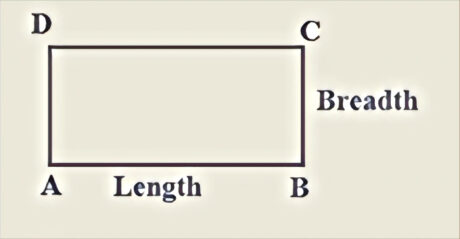#### The perimeter of regular shapes

The perimeter of a triangle = sum of all it’s sides

###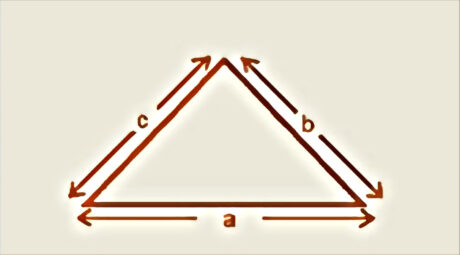The perimeter of a square = 4 (length of a side)

###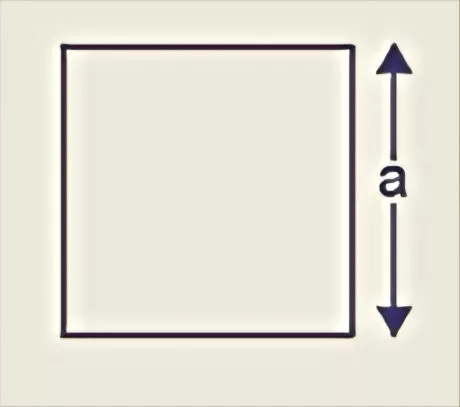If the triangle  is an equilateral triangle that is all its  sides are equal (a = b = c),

then its perimeter is equal to 3( length of a side)

There is a fascinating similarity between a square and an equilateral triangle. They are figures having the sides of equivalent length and equivalent angles. Such figures are called regular closed figures. So, a square and an equilateral triangle are regular closed figures.

#### The perimeter of a polygon

The perimeter of an ‘n’ sided polygon

A polygon is a closed formed with different line segments.

The perimeter of ‘n’ sided polygon = n× length of one side.

For instance: Length of each side of a hexagon is ‘a’ cm, at that point:

Perimeter of the hexagon = 6(a) cm

#### Area

The measure of the surface enclosed by a closed figure is called its Area.

The comparison of two figures regarding which one has a bigger region is hard to make just by taking a look at these figures. To fathom the reason, we put the figure on a squared paper or diagram paper whose each square measures 1 cm × 1 cm. Outline the figure. Take a look at the squares encased by the figure. Some of them are totally encased, some a large portion of, some not exactly half and some the greater part. To defeat this trouble, the accompanying show is embraced:

The area of one complete square is taken as 1 square unit. On a centimetre square sheet, the area of one complete square will be 1 sq cm.

Overlook part of the area that is less than half a square.

Count more than half of the square in a region as one full square.

And take the exact half of the square in a region as sq unit.

At the last, the area of the figure is the number of centimetre squares that are needed to cover it.

The area of a rectangle = Length × Breadth

###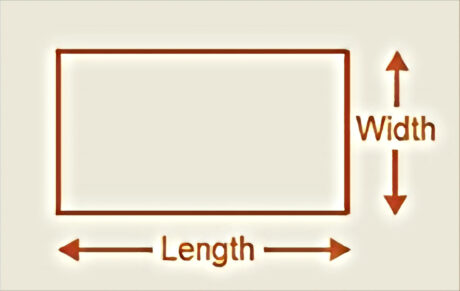Area of a square = Side × Side.

Area of triangle is 1/2 (base)(height)

###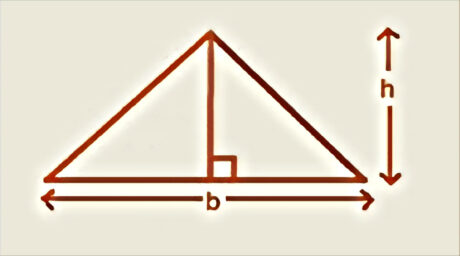#### Visualisation of Area

In the given chart, in the event that the area of every little square is 1 cm2, at that point

Area of square shape = length(5)× breath(2)  = 10cm2

The area of square = side(2)× side(2)  =4cm2

###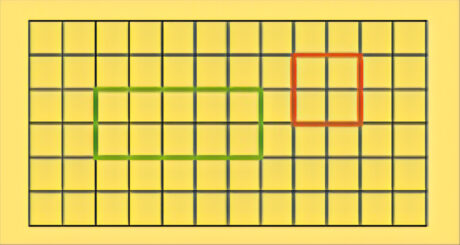#### Area of irregular shapes

Area of an irregular figure can be determined as follows :

Step 1: Divide the irregular shape into ordinary shapes that are known(eg. triangles, square shapes, circles and squares)

Step 2: Add the area of these individual shapes. The total will be the area of the irregular figure.

Below is salved example.

### Questions:

Question:  Explain what is a plane figure?

Answer: It alludes to a level 2D (two-dimensional) shape. Also, they can be made of straight lines, curved lines, or both curved and straight lines. Moreover, plane figures, for example, square and square shape have length and breadth yet other plane figures don’t have length and breadth.

Question: What are the geometric figures?

Answer: These are the figures that we find in math, and they have an alternate name. For example, circle, trapezium, triangle, rectangle, square, heart, and so forth are a few of the geometric shapes. Be that as it may, a few of the shapes, for example, polygons have been depicted dependent on their number of sides.

Question: Are cones and cylinders plane figures?

Answer: No, the cone is a strong three-dimensional figure that has a round base and one vertex. Plus, a cylinder is a solid figure with two equal consistent bases. In any case, a plane figure is level two-dimensional figures or shapes.

October 29, 2020